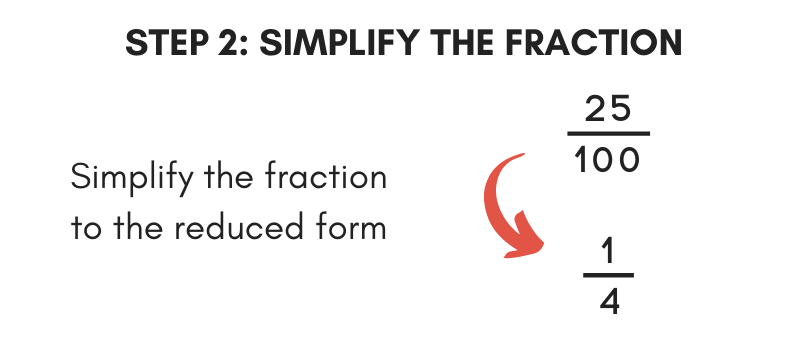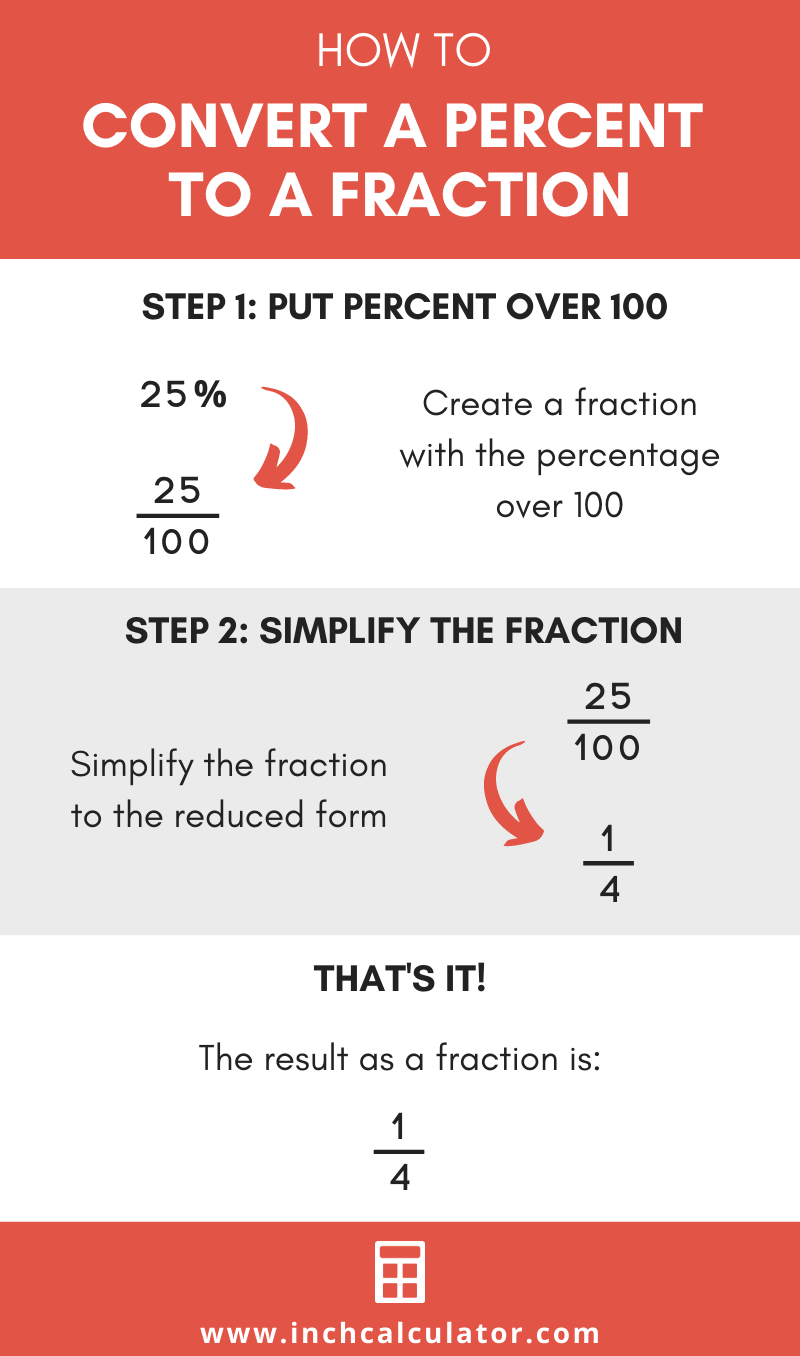# Percent to Fraction Calculator

Enter a percentage below to convert it to a fraction. The calculator shows all of the work so you can see the steps used.

%

## Fraction:

1 / 4

### Steps to Convert Percentage to Fraction

Step One: Convert percentage to a decimal number
25%=0.25
Step Two: Convert from a decimal to a fraction
0.25=1 / 4
We explain this step in more detail on our decimal to fraction calculator
Learn how we calculated this below

Do you want to convert a fraction to percent?

## How to Convert a Percent to a Fraction

There are a few ways to convert a percentage to fraction form. One way is to use a calculator like the one above. Follow along to learn two more methods.

### Method One: Put the Percentage in a Fraction Over 100

The most common method to convert a percent to a fraction is to put the percentage directly into a fraction as the top number over 100. Be sure to remove the percent symbol.

Note that if the top number of the fraction isn’t a whole number, you can multiply the top and bottom numbers by 10 until the top number is a whole number.The final step is to simplify the fraction by reducing. This step is only required if the fraction is not already in the simplest form.For example, convert 25% to a fraction by putting it in a fraction over 100.

25% = 25 / 100

Then simplify
25 / 100 = 1 / 4

Thus, the fraction value of 25% is 1 / 4

### Method Two: Convert to a Decimal and Then to a Fraction

One way to convert a percent value to a fraction is to convert the percentage to decimal form, and then convert the decimal value to a fraction.

To convert to a decimal, divide the percent value by 100. You should also remove the percent symbol from the decimal number.

To complete the conversion, change the decimal number into a fraction. To do this, place the decimal number as the top number of the fraction over 1.

If the decimal is not a whole number, then multiply the top and bottom of the fraction by 10 until the top number is a whole number.

Then simplify the fraction to get the final solution.

For example, convert 25% to a fraction using this method.

Convert to decimal form.
25% = 25 ÷ 100
25% = .25

Convert to fraction form.
.25 = .25 / 1
.25 = (.25 × 10) / (1 × 10)
.25 = (2.5 × 10) / (10 × 10)
.25 = 25 / 100

Then simplify
25 / 100 = 1 / 4

## Percent to Fraction Conversion Chart

Refer to the following percentage to fraction conversion table to see the fractions equal to common percent values.

Percent to fraction conversion table showing common percentages and their equivalent fraction values.
Percent Fraction
5% 1/20
10% 1/10
15% 3/20
20% 1/5
25% 1/4
30% 3/10
35% 7/20
40% 2/5
45% 9/20
50% 1/2
55% 11/20
60% 3/5
65% 13/20
70% 7/10
75% 3/4
80% 4/5
85% 17/20
90% 9/10
95% 19/20
100% 1/1